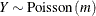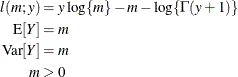# The NLMIXED Procedure

### Built-in Log-Likelihood Functions

This section displays the basic formulas used by the NLMIXED procedure to compute the conditional log-likelihood functions of the data given the random effects. Note, however, that in addition to these basic equations, the NLMIXED procedure employs a number of checks for missing values and floating-point arithmetic. You can see the entire program used by the NLMIXED procedure to compute the conditional log-likelihood functions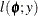by adding the LIST debugging option to the PROC NLMIXED statement.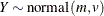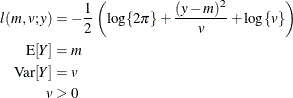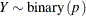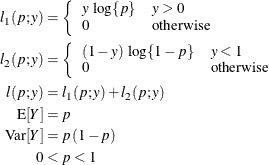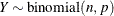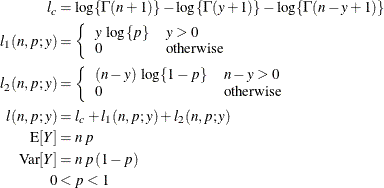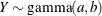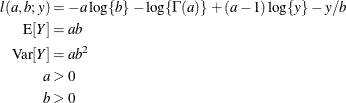This parameterization of the gamma distribution differs from the parameterization used in the GLIMMIX and GENMOD procedures. The following statements show the equivalent reparameterization in the NLMIXED procedure that fits a generalized linear model for gamma-distributed data in the parameterization of the GLIMMIX procedure:

proc glimmix;
model y = x / dist=gamma s;
run;


proc nlmixed;
parms b0=1 b1=0 scale=14;
linp = b0 + b1*x;
mu   = exp(linp);
b    = mu/scale;
model y ~ gamma(scale,b);
run;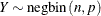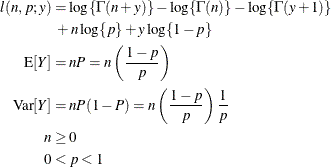This form of the negative binomial distribution is one of the many parameterizations in which the mass function or log-likelihood function appears. Another common parameterization uses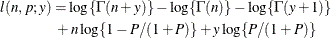with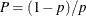,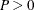.

Note that the parameter n can be real-numbered; it does not have to be integer-valued. The parameterization of the negative binomial distribution in the NLMIXED procedure differs from that in the GLIMMIX and GENMOD procedures. The following statements show the equivalent formulations for maximum likelihood estimation in the GLIMMIX and NLMIXED procedures in a negative binomial regression model:

proc glimmix;
model y = x / dist=negbin s;
run;


proc nlmixed;
parms b0=3, b1=1, k=0.8;
linp = b0 + b1*x;
mu = exp(linp);
p  = 1/(1+mu*k);
model y ~ negbin(1/k,p);
run;